Question

# Suppose a random sample of size 64 is selected from a population. The sample yields a...

Suppose a random sample of size 64 is selected from a population. The sample yields a mean of 26 and a standard deviation of 4. From this information, the 90% confidence interval to estimate the population mean can be computed to be _______.

Solution :

Given that,

Point estimate = sample mean =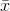= 26

Population standard deviation =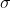= 4

Sample size = n =64

At 90% confidence level the z is ,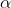= 1 - 90% = 1 - 0.90 = 0.1/ 2 = 0.1 / 2 = 0.05

Z/2 = Z0.05 = 1.645 ( Using z table )

Margin of error = E = Z/2 * (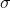/n)

= 1.645 * ( 4 /64 )

= 0.8225
At 90% confidence interval estimate of the population mean
is,- E <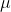<+ E

26 - 0.8225 << 26 + 0.8225

25.1775 << 26.8225

#### Earn Coins

Coins can be redeemed for fabulous gifts.

Similar Homework Help Questions
• ### Suppose that a random sample of size 64 is to be selected from a population with...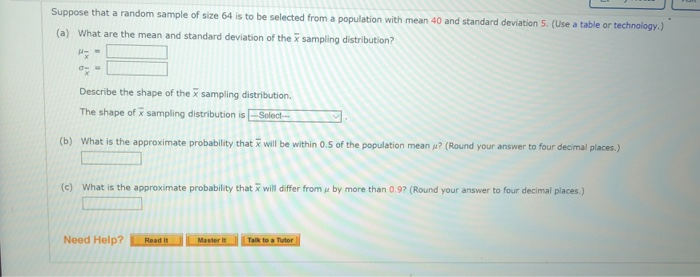Suppose that a random sample of size 64 is to be selected from a population with mean 40 and standard deviation 5. (Use a table or technology.) (a) What are the mean and standard deviation of the x sampling distribution? Describe the shape of the x sampling distribution. The shape of sampling distribution is-Select- (b) What is the approximate probability that will be within 0.5 of the population mean? (Round your answer to four decimal places.) (c) What is the...

• ### Suppose that a random sample of size 64 is to be selected from a population with...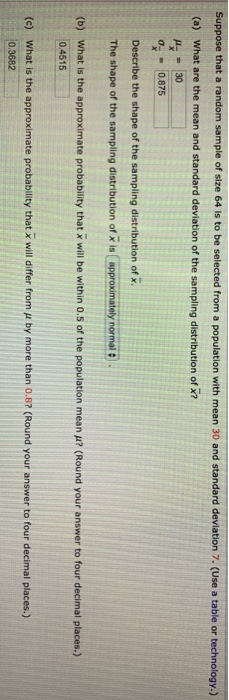Suppose that a random sample of size 64 is to be selected from a population with mean 30 and standard deviation 7. (Use a table or technology.) (a) What are the mean and standard deviation of the sampling distribution of x? - 30 0 - 0.875 Describe the shape of the sampling distribution of x. The shape of the sampling distribution of x is approximately normale (b) What is the approximate probability that x will be within 0.5 of the...

• ### Suppose a random sample of 36 is selected from a population with a standard deviation of...

Suppose a random sample of 36 is selected from a population with a standard deviation of 12. If the sample mean is 98, the 99% confidence Interval to estimate the population mean is what.

• ### you take a random sample size of 1500 from population 1 and a random sample size...

you take a random sample size of 1500 from population 1 and a random sample size of 1500 from population two. the mean of the first sample size is 76; the sample standard deviation is 20. the mean of the second sample is 62; the sample standard deviation is 18. construct the 90% confidence interval estimate of the difference between the means of the two populations representwd here and report both the upper and lower bound of the interval.

• ### 1. A random sample of 24 values was selected from a population, and the sample standard...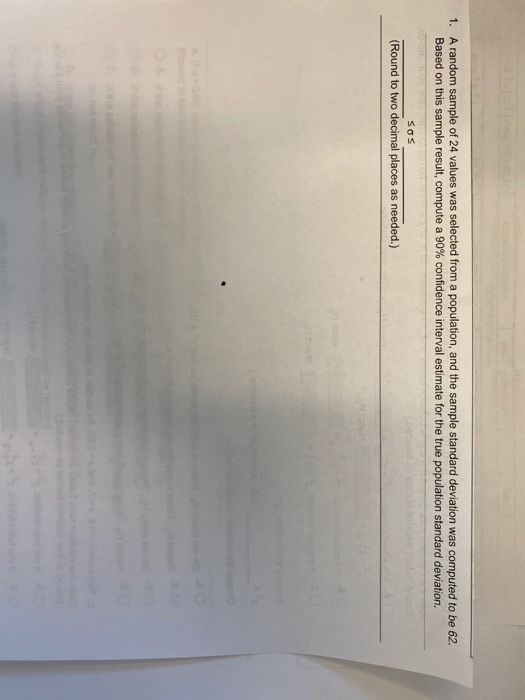1. A random sample of 24 values was selected from a population, and the sample standard deviation was computed to be 62. Based on this sample result, compute a 90% confidence interval estimate for the true population standard deviation. SOS (Round to two decimal places as needed.)

• ### A simple random sample of size 64 is drawn from a normal population with a known...

A simple random sample of size 64 is drawn from a normal population with a known standard deviation σ. The 95% confidence interval for the population mean μ is found to be (12, 16). The approximate population standard deviation σ is:

• ### Suppose that a random sample of size 64 is to be selected from a population with mean 40 and standard deviation 5.

Suppose that a random sample of size 64 is to be selected from a population with mean 40 and standard deviation 5. (a) What are the mean and standard deviation of the sampling distribution? (b) What is the approximate probability that will be within 0.3 of the population mean u? (Round your answer to four decimal places.) (c) What is the approximate probability that will differ from u by more than 0.7? (Round your answer to four decimal places.)

• ### A random sample of size n = 64 is selected from a population with mean μ...

A random sample of size n = 64 is selected from a population with mean μ = 52 and standard deviation σ = 24. a. What will be the approximate shape of the sampling distribution of x? skewed symmetric normal b. What will be the mean and standard deviation of the sampling distribution of x? mean= standard deviation=

• ### Suppose a random sample of size 17 was taken from a normally distributed population, and the...

Suppose a random sample of size 17 was taken from a normally distributed population, and the sample standard deviation was calculated to be s = 5.0. a) Calculate the margin of error for a 95% confidence interval for the population mean. Round your response to at least 3 decimal places.     b) Calculate the margin of error for a 90% confidence interval for the population mean. Round your response to at least 3 decimal places.

• ### Suppose that a sample of size 10 is selected from a population with unknown variance. If...

Suppose that a sample of size 10 is selected from a population with unknown variance. If this information is used to construct a confidence interval for a single population mean, which of the following statements is true? Select the best answer. The sample of size 10 must be normally distributed. The standard normal distribution must be used to construct the confidence interval. The sample mean cannot be used as a point estimate for the population mean because the sample size...